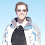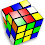## Friday, May 16, 2008

### Googol to the Googol-th Power

At this point everyone should be familiar with the number "googol" which is 10^100 (10 to the 100th power). Written down it is a one followed by 100 zeroes.

The question this week is:
Q: How many zeroes are there in googol^googol
(googol to the "googol-th" power)?

1.One hundred googol zeros.

2.Eric is right. To figure the number of zeroes, just take the common log:

log(googol^googol)

Use this rule: log(a^b) = b log(a)
= googol * log(googol)
= googol * log(10^100)

Use the rule again:
= googol * 100 * log(10)

Finally log(10) is simply 1:
= 100 googol * 1
= 100 googol